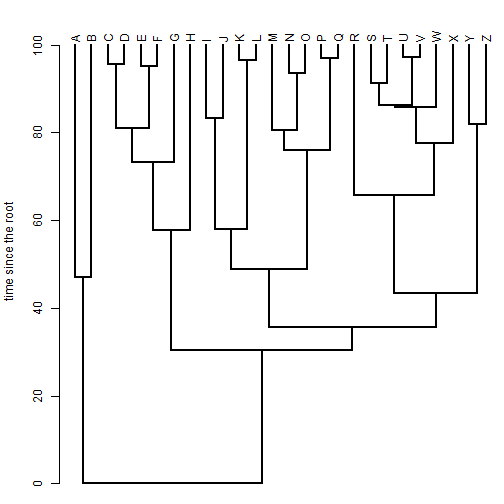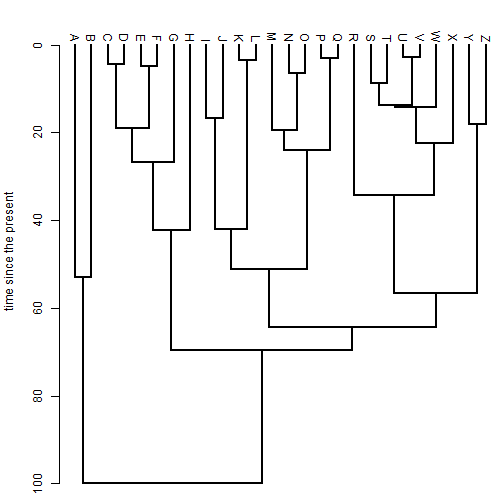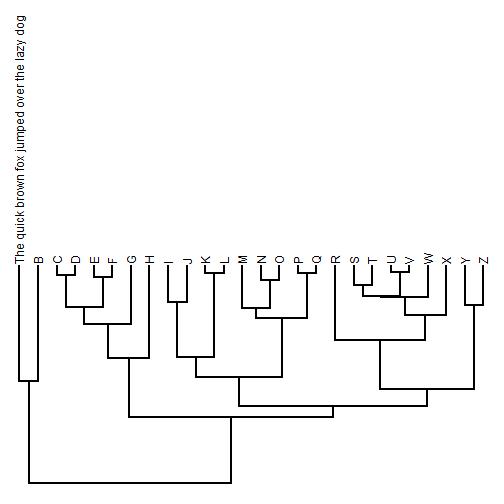## Sunday, February 12, 2017

### Plotting up- & down-facing phylograms in `phytools::plotSimmap`

I just pushed a significant update to the workhorse phytools plotting function `plotSimmap` to permit plotting in both up- and down-facing square phylograms, in addition to the right & left phylograms and the circular fan trees already available.

When I call `plotSimmap` a “workhorse,” what I mean is that it is used internally by a whole bunch of other functions & methods in the package. For instance, in addition to the S3 plot method for `"simmap"` and `"multiSimmap"` object classes, it is also used by `bind.tip` (in interactive mode), `contMap` (& `plot.contMap`), `densityMap` (& `plot.densityMap`), `densityTree`, `dotTree` (for only one character), `fancyTree` (for various methods), `ltt`, `plot.describe.simmap`, `plotTree`, `plotTree.wBars` (along with both `plotTree.barplot`, and `plotTree.boxplot`), `reroot` (for interactive mode), and `treeSlice` (in `prompt=TRUE` mode), among other things. Adding the up- & down-facing directions to this method will eventually allow me to add this capability to many of these functions as well.

`plotSimmap` is also used inside the new functions of the physketch package, such as in the `phylo.tracer` function to draw a `"phylo"` object on top of the image of a plotted tree. When I extend the `direction` argument to this function it will be possible to trace a phylogeny plotted in any direction.

Here's a quick demo of up- & down-facing trees:

``````library(phytools)
packageVersion("phytools")
``````
``````##  '0.5.75'
``````
``````plotTree(tree,direction="upwards",mar=c(0.1,4.1,0.1,0.1))
axis(side=2,at=seq(0,100,by=20))
title(ylab="time since the root")
``````We can flip the direction of the axis - that is, plotting time from the present instead of time since the root - by plotting our tree downwards, but then switch `ylim{1]` & `ylim{2]`.

For instance:

``````obj<-get("last_plot.phylo",envir=.PlotPhyloEnv)
ylim<-obj\$y.lim[2:1]-(obj\$y.lim-max(nodeHeights(tree)))
plotTree(tree,direction="downwards",mar=c(0.1,4.1,0.1,0.1),ylim=ylim)
axis(side=2,at=seq(0,100,by=20))
title(ylab="time since the present")
``````One (surprising?) challenge with tree plotting is ensuring that there is enough, but not too much, space left for tip labels. The reason this is such a pain is because we don't know how much space the labels will take up in the units of our tree until we've already plotted the tree! Let's make one crazy long label to see if I got it right this time:

``````tree\$tip.label<-"The quick brown fox jumped over the lazy dog"
plotTree(tree,direction="upwards")
``````There are still some bugs to be worked it before I can add this option to all the other functions listed above - so please be patient.

That's it.